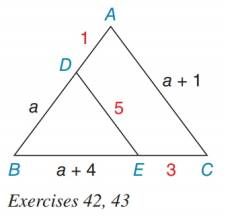Chapter 5.1, Problem 42EElementary Geometry For College St...

7th Edition
Alexander + 2 others
ISBN: 9781337614085

Solutions

Chapter
SectionElementary Geometry For College St...

7th Edition
Alexander + 2 others
ISBN: 9781337614085
Textbook Problem

In the figure, assume that a > 0. Prove that B D B A ≠ B E B C .To determine

To prove:

BDBABEBC.

Explanation

It is given, BD = a, DA = 1, BE = a + 4 and EC = 3.

Find BA and BC using BD = a, DA = 1, BE = a + 4 and EC = 3.

BA = BD + DA

= a + 1

BC= BE + EC

= a + 4 + 3

= a + 7

Substitute BD = a and BA = a + 1 in BDBA.

BDBA=aa+1     .....(1)

Substitute BE = a + 4 and BC = a + 7 in BEBC.

BEBC=a+4a+7

Still sussing out bartleby?

Check out a sample textbook solution.

See a sample solution

The Solution to Your Study Problems

Bartleby provides explanations to thousands of textbook problems written by our experts, many with advanced degrees!

Get Started

Simplify the expressions in Exercises 97106. x3/2x5/2

Finite Mathematics and Applied Calculus (MindTap Course List)

In Exercises 75-98, perform the indicated operations and/or simplify each expression. 94. (x2 1)(2x2 x2(2x)

Applied Calculus for the Managerial, Life, and Social Sciences: A Brief Approach

Solve the differential equation. 7. 2yey2y=2x+3x

Single Variable Calculus: Early Transcendentals

For f(x) = tanh1 2x, f(x) = a) 2(sech1 2x)2 b) 2(sech2 2x)1 c) 214x2 d) 114x2

Study Guide for Stewart's Single Variable Calculus: Early Transcendentals, 8th

For , f′(x) =

Study Guide for Stewart's Multivariable Calculus, 8th